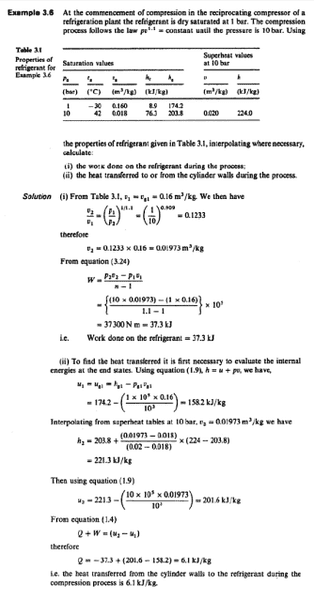# Thermodynamics Polytropic Process Compressor

I was revising for my course exam and encountered a question in my book (Applied Thermodynamics for Engineering Technologists, p 66 example 3.3) which stated that a compressor follows a polytropic process to compress air; I understood the calculation process which resulted in heat being transferred to the refrigerant. The problem is that I cannot understand how physically a compressor would be absorbing heat from the environment. I always thought that any type of compression would give off heat.

Theoretically I know that for a polytropic process if n=γ the process is adiabatic, if n is smaller than γ heat is given off and if n is bigger than γ heat is received into the working gas (), but I would like to know how this is physically possible.

The question I talked about above is the one in the image below.It never went cross my eyes that n has anything to do with heat absorption and heat rejection. Simple is that, when a gas is compressed above its critical temp, its temperature increases. You are giving energy in the form of electricity which is converted into mechanical energy by a piston or impeller,this increases kinetic energy of the fluid.where would this energy go? It would increase its temp.

According to the above problem, may be the surrounding temperature is above refrigerant‘s temperature.which caused heat transfer.

:-)
Thanks

According to the above problem, may be the surrounding temperature is above refrigerant‘s temperature.which caused heat transfer.

Maybe that is true, but I'm not sure about it. Thanks :)

Energy is transfered to a system either by work or by heat transfer,in above problem they have already found out the portion of energy tranfered by work,rest is heat transfer,which is obviously due to temperature difference.since Q is positive,it means heat is added to the system,thus surrounding/casing temp was greater then refrigerant temp.

Energy is transfered to a system either by work or by heat transfer,in above problem they have already found out the portion of energy tranfered by work,rest is heat transfer,which is obviously due to temperature difference.since Q is positive,it means heat is added to the system,thus surrounding/casing temp was greater then refrigerant temp.

Oh, okay I understood the idea now. Seems so simple after the explanation, I was blind folded by the fact that a compression always releases heat. Thanks for your input :)